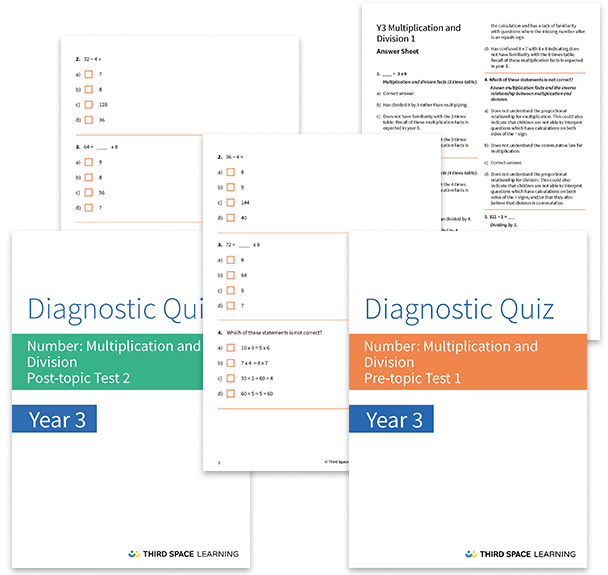# Multiplication and Division Diagnostic Assessments Year 3

This pack of resources contains two diagnostic assessments for multiplication and division, one pre and one post topic assessment. Each test contains 20 multiple choice questions with detailed answers that not only indicate the correct answer but also explain the distractor answers. You can use these tests to help you diagnose specific misconceptions and learning gaps before starting a block of learning and after the block has been completed.

These tests cover:

• The 3, 4 and 8 times tables (including multiplication and division facts)
• Understanding the inverse relationship between multiplication and division
• Dividing by 1
• Multiplying a multiple of 10 by a one-digit number
• Multiplying and dividing a two-digit number by a one-digit number (including with remainders)
• Scaling problems

Year 3

Multiplication, Division